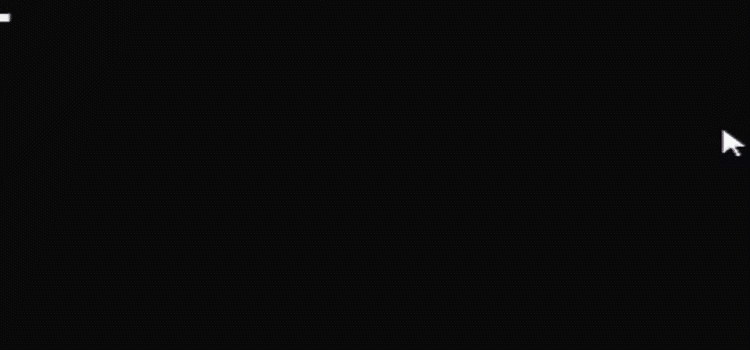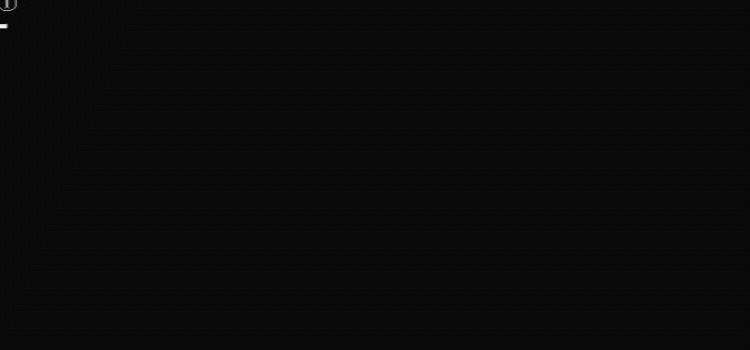# C語言實現彈跳小球項目

## 一、項目描述和最終項目展示## 二、輸出一個小球

```#include<stdio.h>
//靜止的小球
int main(void)
{
int i,j;
for(i=0;i<6;i++)
printf("\n");
for(j=0;j<6;j++)
printf(" ");
printf("①\n");
return 0;
}```## 三、下落的小球

```//下降的小球
#include<stdio.h>
#include<windows.h>
int main(void)
{
int i,j;
int x,y;
int sum=0;
x=10;
y=10;
for(x=1;x<10;x++)//控制行數，即下落的距離
{
system("cls");
sum=0;
for(i=0;i<x;i++)//輸出行數
{
printf("\n");
sum++;
}
for(j=0;j<y;j++)//距離原點的橫著的距離
{
printf(" ");
}
printf("①\n");
}
return 0;
}```## 四、來回彈跳的小球

```//上下彈跳的小球
#include<stdio.h>
#include<stdlib.h>
int main(void)
{
int i,j;
int x=0;
int y=10;

int height=20;//最大高度
int velocity=1;//你的加速度
while(1)
{
x=x+velocity;//高度是越來越高
system("cls");

//輸出小球前的空行
for(i=0;i<x;i++)
{
printf("\n");
}
for(j=0;j<y;j++)
{
printf(" ");
}
printf("①");
printf("\n");
if( x == height )//到底的時候，加速度變為負數
velocity = -velocity;
if( x == 0 )//到頂的時候，加速度變為正數
velocity = -velocity;
}
}```## 五、最終項目實現

```#include<stdio.h>
#include<stdlib.h>
int main(void)
{
int i,j;
int x=0;
int y=0;

int velocity_x=1;//x加速度
int velocity_y=1;//y加速度

int left=0;//左邊的邊
int right=20;//右邊的邊
int top=0;//頂部
int bottom=10;//底部

while(1)
{
x = x+velocity_x;
y = y+velocity_y;

system("cls");
for(i=0;i<x;i++)
{
printf("\n");
}
for(j=0;j<y;j++)
{
printf(" ");
}
printf("①");
printf("\n");

if( (x==top) || (x==bottom) )
//加速度正負的變化
{
velocity_x=-velocity_x;
}
if( (y==left) || (y==right) )
{
velocity_y=-velocity_y;
}
}
}```